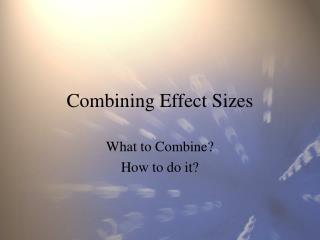Download PresentationCombining Effect Sizes

# Combining Effect Sizes - PowerPoint PPT PresentationDownload Presentation## Combining Effect Sizes

- - - - - - - - - - - - - - - - - - - - - - - - - - - E N D - - - - - - - - - - - - - - - - - - - - - - - - - - -
##### Presentation Transcript

1. Combining Effect Sizes What to Combine? How to do it?

2. What to Combine? (1) • Whether effect sizes can/should be combined is controversial • Safe if studies are replications • (identical IV, DV, design, only difference is observations) • Random samples from same population – results in a sampling distribution

3. What to Combine (2) • Not safe or reasonable to combine if • IV or DV measures different constructs across studies (e.g., DV in study 1 is taxes paid, and DV in study 2 is subjective well being) • Different study designs ( study 1 has random assignment to condition; study 2 allows participants to choose conditions)

4. What to Combine (3) • OK to combine if • Measures are same across studies (e.g., all studies use GRE) (can use unstandardized ES in analysis) • Measures across all studies are parallel (can use unstandardized ES) • Measures are essentially tau equivalent (same reliability, different mean and SD); use Standardized ES • Measures are congeneric (diff reliability, different M and SD) use Standardized ES, may adjust model for reliability.

5. What to Combine (4) • Most social science studies are hard to justify as proper for a meta-analysis • Glass (any and all therapy, any and all outcomes) • Gaugler (assessment centers) • When is a study a replication? • Compare to medicine (e.g., aspirin and heart attack)

6. How to Combine (1) • Take the simple mean (add all ES, divide by number of ES) M=(1+.5+.3)/3 M = 1.8/3 M=.6 Unbiased, consistent, but not efficient estimator.

7. How to Combine (2) • Take a weighted average M=(1+1+.9)/(1+2+3) M=(2.9)/6 M=.48 (cf .6 w/ unit wt) (Unit weights are special case where w=1.)

8. How to Combine (3) • Choice of Weights (all are consistent, will give good estimates as the number of studies and sample size of studies increases) • Unit • Unbiased, inefficient • Sample size • Unbiased (maybe), efficient relative to unit • Inverse variance – endorsed by PMA • Reciprocal of sampling variance • Biased (if parameter figures in sampling variance), most efficient • Other – special weights depend on model, e.g., adjust for reliability (Schmidt & Hunter)

9. How to Combine (4) • Inverse Variance Weights are a function of the sample size, and sometimes also a parameter. • For the mean: • For r: • For r transformed to z: Note that for two of three of these, the parameter is not part of the weight. For r, however, larger observed values will get more weight. Mean can be biased.